International
Tables for
Crystallography
Volume A
Space-group symmetry
Edited by M. I. Aroyo

International Tables for Crystallography (2016). Vol. A, ch. 1.6, pp. 114-126

## Section 1.6.5.1. Applications of resonant scattering to symmetry determination

H. D. Flackb

| top | pdf |

#### 1.6.5.1.1. Introduction

| top | pdf |

In small-molecule crystallography, it has been customary in crystal-structure analysis to make no use of the contribution of resonant scattering (otherwise called anomalous scattering and in older literature anomalous dispersion) other than in the specific area of absolute-structure and absolute-configuration determination. One may trace the causes of this situation to the weakness of the resonant-scattering contribution, to the high cost in time and labour of collecting intensity data sets containing measurements of all Friedel opposites and for a lack of any perceived or real need for the additional information that might be obtained from the effects of resonant scattering.

On the experimental side, the turning point came with the widespread distribution of area detectors for small-molecule crystallography, giving the potential to measure, at no extra cost, full-sphere data sets leading to the intensity differences between Friedel opposites hkl and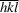. In 2015, the new methods of data analysis briefly presented here are in the stage of development and have not yet enjoyed widespread distribution, use and acceptance by the community. Flack et al. (2011) and Parsons et al. (2012) give detailed information on these calculations.

#### 1.6.5.1.2. Status of centrosymmetry and resonant scattering

| top | pdf |

The basic starting point in this analysis is the following linear transformation ofand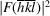, applicable to both observed and model values, to give the average (A) and difference (D) intensities: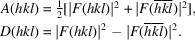In equation (1.6.2.1),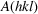was denoted by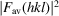. The expression for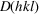corresponding to that forgiven in equation (1.6.2.1)and using the same nomenclature is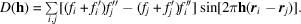In general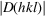is small compared to. A compound with an appreciable resonant-scattering contribution has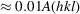, whereas a compound with a small resonant-scattering contribution has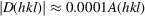. For centric reflections,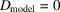, and so the values of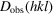of these are entirely due to random uncertainties and systematic errors in the intensity measurements.of acentric reflections contains contributions both from the random uncertainties and the systematic errors of the data measurements, and from the differences betweenandwhich arise through the effect of resonant scattering. A slight experimental limitation is that a data set of intensities needs to contain both reflections hkl andin order to obtain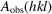and.

The Bijvoet ratio, defined by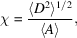is the ratio of the root-mean-square value of D to the mean value of A. In a structure analysis, two independent estimates of the Bijvoet ratio are available and their comparison leads to useful information as to whether the crystal structure is centrosymmetric or not.

The first estimate arises from considerations of intensity statistics leading to the definition of the Bijvoet ratio as a value called Friedifstat, whose functional form was derived by Flack & Shmueli (2007) and Shmueli & Flack (2009). One needs only to know the chemical composition of the compound and the wavelength of the X-radiation to calculate Friedifstat using various available software.

The second estimate of the Bijvoet ratio, Friedifobs, is obtained from the observed diffraction intensities. One problematic point in the evaluation of Friedifobs arises because A and D do not have the same dependence on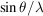and it is necessary to eliminate this difference as far as possible. A second problematic point in the calculation is to make sure that only acentric reflections of any of the noncentrosymmetric point groups in the chosen Laue class are selected for the calculation of Friedifobs. In this way one is sure that if the point group of the crystal is centrosymmetric, all of the chosen reflections are centric, and if the point group of the crystal is noncentrosymmetric, all of the chosen reflections are acentric. The necessary selection is achieved by taking only those reflections that are general in the Laue group. To date (2015), the calculation of Friedifobs is not available in distributed software. On comparison of Friedifstat with Friedifobs, one is able to state with some confidence that:

 (1) if Friedifobs is much lower than Friedifstat, then the crystal structure is either centrosymmetric, and random uncertainties and systematic errors in the data set are minor, or noncentrosymmetric with the crystal twinned by inversion in a proportion close to 50:50; (2) if Friedifobs is close in value to Friedifstat, then the crystal is probably noncentrosymmetric and random uncertainties and systematic errors in the data set are minor. However, data from a centrosymmetric crystal with large random uncertainties and systematic errors may also produce this result; and (3) if Friedifobs is much larger than Friedifstat then either the data set is dominated by random uncertainties and systematic errors or the chemical formula is erroneous.

#### Example 1

The crystal of compound Ex1 (Udupa & Krebs, 1979) is known to be centrosymmetric (space group) and has a significant resonant-scattering contribution, Friedifstat = 498 and Friedifobs = 164. The comparison of Friedifstat and Friedifobs indicates that the crystal structure is centrosymmetric.

#### Example 2

The crystal of compound Ex2, potassium hydrogen (2R,3R) tartrate, is known to be enantiomerically pure and appears in space group. The value of Friedifobs is 217 compared to a Friedifstat value of 174. The agreement is good and allows the deduction that the crystal is neither centrosymmetric, nor twinned by inversion in a proportion near to 50:50, nor that the data set is unsatisfactorily dominated by random uncertainty and systematic error.

#### Example 3

The crystals of compound Ex3 (Zhu & Jiang, 2007) occur in Laue group. One finds Friedifstat = 70 and Friedifobs = 499. The huge discrepency between the two shows that the observed values of D are dominated by random uncertainty and systematic error.

#### 1.6.5.1.3. Resolution of noncentrosymmetric ambiguities

| top | pdf |

It was shown in Section 1.6.5.1.2that under certain circumstances it is possible to determine whether or not the space group of the crystal investigated is centrosymmetric. Suppose that the space group was found to be noncentrosymmetric. In each Laue class, there is one centrosymmetric point group and one or more noncentrosymmetric point groups. For example, in the Laue class mmm we need to distinguish between the point groups 222, 2mm, m2m and mm2, and of course between the space groups based on them. We shall show that it is possible in practice to distinguish between these noncentrosymmetric point groups using intensity differences between Friedel opposites caused by resonant scattering.

An excellent intensity data set from a crystal (Ex2 above) of potassium hydrogen (2R, 3R) tartrate, measured with a wavelength of 0.7469 Å at 100 K, was used. The Laue group was assumed to be mmm. The raw data set was initially merged and averaged in point group 1 and all special reflections of the Laue group mmm (i.e. 0kl, h0l, hk0, h00, 0k0, 00l) were set aside. The remaining data were organized into sets of reflections symmetry-equivalent under the Laue group mmm, and only those sets (589 in all) containing all 8 of the mmm-symmetry-equivalent reflections were retained. Each of these sets provides 4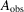and 4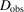values which can be used to calculate Rmerge values appropriate to the five point groups in the Laue class mmm. The results are given in Table 1.6.5.1. The value of 100% for Rmerge in a centrosymmetric point group, such as mmm or 2/m, arises by definition and not by coincidence. The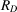of the true point group has the lowest value, which is noticeably different from the other choices of point group.

 Table 1.6.5.1| top | pdf | Rmerge values for Ex2 for the 589 sets of general reflections of mmm which have all eight measurements in the set
Rmerge (%)mmm2mmm2mmm2222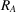1.30 1.30 1.30 1.30 1.30100.0 254.4 235.7 258.1 82.9

The crystal of Ex1 above (space group) was treated in a similar manner. Table 1.6.5.2shows thatvalues display no preference between the three point groups in Laue class 2/m.

 Table 1.6.5.2| top | pdf | Rmerge values for Ex1 for the 724 sets of general reflections of 2/m which have all four measurements in the set
Rmerge (%)2/mm21.29 1.29 1.29100.0 98.3 101.7

Intensity measurements comprising a full sphere of reflections are essential to the success of the Rmerge tests described in this section.

#### 1.6.5.1.4. Data evaluation after structure refinement

| top | pdf |

There is an excellent way in which to evaluate both data measurement and treatment procedures, and the fit of the model to the data, including the space-group assignment, at the completion of structure refinement. This technique is applicable both to noncentrosymmetric and to centrosymmetric crystals. A scattergram of Dobs against Dmodel, and 2Aobs against 2Amodel pairs are plotted on the same graph. All (Dobs, Dmodel) pairs are plotted together with those (2Aobs, 2Amodel) pairs which have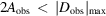. The range of values on the axes of the model and of the observed values should be identical. For acentric reflections, for both A and D, a good fit of the observed to the model quantities shows itself as a straight line of slope 1 passing through the origin, with some scatter about this ideal straight line. For an individual reflection, 2A and D are, respectively, the sum and the difference of the same quantities and they have identical standard uncertainties. It is thus natural to select 2A and D to plot on the same graph. In practice one sees that the spread of the 2A plot increases with increasing value of 2A. Fig. 1.6.5.1shows the 2AD plot for Ex2 of Example 2 in Section 1.6.5.1.2, which is most satisfactory and confirms the choice of point group from the use of Rmerge. The conventional R value for all reflections is 3.1% and for those shown in Fig. 1.6.5.1it is 10.4%. The R value for all D values is good at 51.1%. Fig. 1.6.5.2shows the 2AD plot for Ex1 of Example 1 in Section 1.6.5.1.2. The structure model is centrosymmetric so all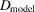values are zero. The conventional R value on A for all reflections is 4.3% and for those shown in Fig. 1.6.5.2it is 9.1%. The R value on all the D values is 100%.Figure 1.6.5.1 | top | pdf |Data-evaluation plot for crystal Ex2. The plot shows a scattergram of all (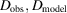) pairs and those (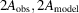) pairs in the same intensity range as the D values.Figure 1.6.5.2 | top | pdf |Data-evaluation plot for crystal Ex1. The plot shows a scattergram of all () and some () data points.

### References

Flack, H. D., Sadki, M., Thompson, A. L. & Watkin, D. J. (2011). Practical applications of averages and differences of Friedel opposites. Acta Cryst. A67, 21–34.
Flack, H. D. & Shmueli, U. (2007). The mean-square Friedel intensity difference in P1 with a centrosymmetric substructure. Acta Cryst. A63, 257–265.
Parsons, S., Pattison, P. & Flack, H. D. (2012). Analyzing Friedel averages and differences. Acta Cryst. A68, 736–749.
Shmueli, U. & Flack, H. D. (2009). Concise intensity statistics of Friedel opposites and classification of the reflections. Acta Cryst. A65, 322–325.
Udupa, M. R. & Krebs, B. (1979). Crystal and molecular structure of creatininium tetrachlorocuprate(II). Inorg. Chim. Acta, 33, 241–244.
Zhu, H.-Y. & Jiang, S.-D. (2007). 1,3,4,6-Tetra-O-acetyl-2-(trifluoromethylsulfonyl)-β-D-mannopyranose. Acta Cryst. E63, o2833.